# A motivating example: descriptive epidemiological meta-regression of Parkinson's Disease¶

The goal of this document it give a concise demonstration of the strengths and limitations of DisMod-MR, the descriptive epidemiological meta-regression tool developed for the Global Burden of Disease, Injuries, and Risk Factors 2010 (GBD2010) Study.

A systematic review of PD was conducted as part of the GBD 2010 Study. The results of this review---data on the prevalence, incidence, and standardized mortality ratio of PD---needed to be combined to produce estimates of disease prevalence by region, age, sex, and year. These prevalence estimates were combined with disability weights to measure years lived with disability (YLDs), which were then combined with estimates of years of life lost (YLLs) to produce estimates of the burden of PD quantified in disability-adjusted life-years (DALYs).

PD is a neurodegenerative disorder that includes symptoms of motor dysfunction, such as tremors, rigidity, and akinesia, in the early stages of the disease. As the disease develops, most patients also develop nonmotor symptoms, such as cognitive decline, dementia, autonomic failure, and disordered sleep-wake regulation. The standard definition for PD diagnosis includes at least two of four cardinal signs---resting tremor, bradykinesia, rigidity, and postural abnormalities. There is no cure or treatments to slow the progression of the disease; however, motor symptoms and disability may be improved with symptomatic therapy.

This document works with simulated data, so that the dataset is fully distributable. This data is included for understanding how to use DisMod-MR, and is not intended for the study of the descriptive epidemiology of PD.

In :
import numpy as np, matplotlib.pyplot as plt, pandas as pd, pymc as mc
import dismod_mr


DisMod-MR uses the integrative systems modeling (ISM) approach to produce simultaneous estimates of disease incidence, prevalence, remission, and mortality. The hallmark of ISM is incorporating all available data. In the case of Parkinson's Disease this consists of population level measurements of incidence, prevalence, standardized mortality rate (SMR), and cause-specific mortality rate (CSMR).

I will begin with a look at a subset of this data, however. Only that from females in the Europe, Western GBD region.

In :
model = dismod_mr.data.load('pd_sim_data')
model.keep(areas=['europe_western'], sexes=['female', 'total'])

kept 348 rows of data


Of the 348 rows of data, here is how the values breakdown by data type:

In :
summary = model.input_data.groupby('data_type')['value'].describe()
np.round(summary,3).sort_values('count', ascending=False)

Out:
count mean std min 25% 50% 75% max
data_type
p 226.0 0.005 0.008 0.000 0.000 0.003 0.007 0.052
m_all 44.0 0.060 0.125 0.000 0.000 0.003 0.033 0.500
csmr 39.0 0.000 0.000 0.000 0.000 0.000 0.000 0.001
i 33.0 0.001 0.001 0.000 0.000 0.000 0.001 0.008
smr 6.0 1.762 0.672 1.115 1.276 1.569 2.100 2.864

More than half of the available data for this region is prevalence data. I'll take a closer look at that now.

In :
model.get_data('smr').value.mean()

Out:
1.7620067430983335
In :
groups = model.get_data('p').groupby('area')
print(np.round_(groups['value'].describe(),3).sort_values('50%', ascending=False))

                count   mean    std    min    25%    50%    75%    max
area
AUT               1.0  0.006    NaN  0.006  0.006  0.006  0.006  0.006
ESP              43.0  0.006  0.007  0.000  0.001  0.006  0.009  0.037
europe_western   13.0  0.007  0.006  0.000  0.001  0.006  0.013  0.017
FRA              19.0  0.007  0.008  0.000  0.001  0.005  0.010  0.027
DNK               6.0  0.004  0.003  0.001  0.001  0.004  0.005  0.009
FIN               1.0  0.004    NaN  0.004  0.004  0.004  0.004  0.004
NLD              20.0  0.009  0.015  0.000  0.000  0.004  0.011  0.052
NOR               4.0  0.005  0.004  0.002  0.003  0.003  0.005  0.011
SWE               6.0  0.003  0.003  0.000  0.001  0.003  0.004  0.008
ITA              53.0  0.004  0.006  0.000  0.000  0.002  0.005  0.033
GBR              36.0  0.004  0.006  0.000  0.000  0.001  0.005  0.022
ISR               4.0  0.003  0.004  0.000  0.000  0.001  0.004  0.009
DEU               9.0  0.004  0.009  0.000  0.000  0.000  0.006  0.026
PRT              11.0  0.002  0.003  0.000  0.000  0.000  0.003  0.010


In the original dataset, there was a wide range in median values, which reflects a combination of country-to-country variation and compositional bias. Simulating data has reduced this substantially, but there is still six-fold variation between ESP and GBR.

In :
countries = ['ESP', 'GBR']
c = {}
for i, c_i in enumerate(countries):
c[i] = groups.get_group(c_i)

In :
ax = None
plt.figure(figsize=(10,4))
for i, c_i in enumerate(countries):
ax = plt.subplot(1,2,1+i, sharey=ax, sharex=ax)
dismod_mr.plot.data_bars(c[i])
plt.xlabel('Age (years)')
plt.ylabel('Prevalence (per 1)')
plt.title(c_i)
plt.axis(ymin=-.001, xmin=-5, xmax=105)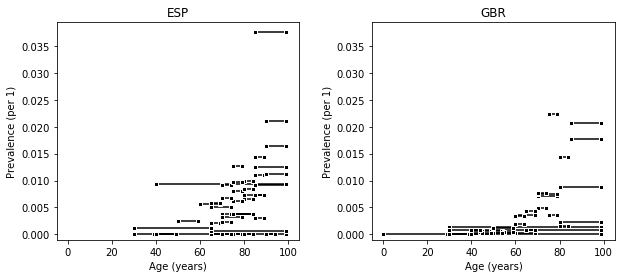## A model for age-specific parameters when measurements have heterogeneous age groups¶

DisMod-MR has four features that make it particularly suited for estimating age-specific prevalence of PD from this data:

• Piecewise linear spline model for change in prevalence as a function of age
• Age-standardizing model of age-group heterogeneity represents the heterogeneous age groups collected in systematic review
• Country-level random effects for true variation in prevalence between countries
• Negative binomial model of data, which provides data-driven estimation of non-sampling error in measurements and elegantly handles measurements of 0

I will now fit the prevalence data with DisMod-MR's age-standardizing negative binomial random effect spline model and compare the estimates to the observed data. Then I will use the results of the fit model to explore the four features listed above.

In :
# remove fixed effects for this example, I will return to them below
model.input_data = model.input_data.filter(regex='(?!x_)')

In :
model.vars += dismod_mr.model.asr(model, 'p')
%time dismod_mr.fit.asr(model, 'p')

WARNING: 5 rows of p data has invalid quantification of uncertainty.
finding initial values

/ihme/homes/abie/.local/lib/python3.6/site-packages/pandas/core/indexing.py:480: SettingWithCopyWarning:
A value is trying to be set on a copy of a slice from a DataFrame.
Try using .loc[row_indexer,col_indexer] = value instead

See the caveats in the documentation: http://pandas.pydata.org/pandas-docs/stable/user_guide/indexing.html#returning-a-view-versus-a-copy
self.obj[item] = s

. . .
finding MAP estimate

finding step covariances estimate

resetting initial values (1)
. . .
resetting initial values (2)

mare: nan
sampling from posterior


/ihme/homes/abie/.local/lib/python3.6/site-packages/numpy/lib/function_base.py:3250: RuntimeWarning: Invalid value encountered in median
r = func(a, **kwargs)

CPU times: user 2min 59s, sys: 532 ms, total: 3min
Wall time: 3min

Out:
(<pymc.NormalApproximation.MAP at 0x7f7466bab358>,
<pymc.MCMC.MCMC at 0x7f7466b8f6d8>)
In :
# plot age-specific prevalence estimates over data bars
plt.figure(figsize=(10,4))

dismod_mr.plot.data_bars(model.get_data('p'), color='grey', label='Simulated PD Data')
pred = dismod_mr.model.predict_for(model, model.parameters['p'], 'all', 'female', 2005,
'GBR', 'female', 2005, 1.,
model.vars['p'], 0., 1.)    # TODO: simplify this method!

hpd = mc.utils.hpd(pred, .05)

plt.plot(np.arange(101), pred.mean(axis=0), 'k-', linewidth=2, label='Posterior Mean')
plt.plot(np.arange(101), hpd[0,:], 'k--', linewidth=1, label='95% HPD interval')
plt.plot(np.arange(101), hpd[1,:], 'k--', linewidth=1)

plt.xlabel('Age (years)')
plt.ylabel('Prevalence (per 1)')
plt.grid()
plt.legend(loc='upper left')

plt.axis(ymin=-.001, xmin=-5, xmax=105);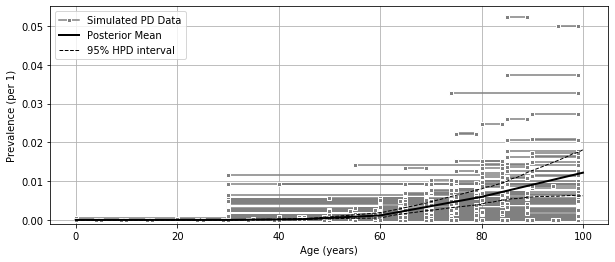In :
p_only = model  # store results for future comparison


This estimate shows the nonlinear increase in prevalence as a function of age, where the slope of the curve increases at age 60. A nonlinear estimate like this is possible thanks to DisMod-MR's piecewise linear spline model.

The age-standardizing model for heterogeneous age groups is also important for such settings; a naive approach, such as using the age interval midpoint, would result in under-estimating the prevalence for age groups that include both individuals older and younger than 60.

The exact age where the slope of the curve changes is not entirely data driven in this example. The knots in the piecewise linear spline model were chosen a priori, on the following grid:

In :
model.parameters['p']['parameter_age_mesh']

Out:
[0, 30, 45, 60, 80, 100]

A sparse grid allows faster computation, but a dense grid allows more expressive age pattens. Choosing the proper balance is one challenge of a DisMod-MR analysis. This is especially true for sparse, noisy data, where too many knots allow the model to follow noisy idiosyncrasies of the data. DisMod-MR allows for penalized spline regression to help with this choice.

The country-level random effects in this model capture country-to-country variation in PD prevalence. This variation is not visible in the graphic above, which shows the regional aggregation of country-level estimates (using a population weighted average that takes uncertainty into account).

The country-level random effects take the form of intercept shifts in log-prevalence space, with values showing in the following:

In :
df = pd.DataFrame(index=[alpha_i.__name__ for alpha_i in model.vars['p']['alpha']],
columns=['mean', 'lb', 'ub'])
for alpha_i in model.vars['p']['alpha']:
trace = alpha_i.trace()
hpd = mc.utils.hpd(trace, .05)
df.loc[alpha_i.__name__] = (np.mean(trace), hpd, hpd)

In :
print(np.round(df.astype(float),3).sort_values('mean', ascending=False))

              mean     lb     ub
alpha_p_GBR  0.033 -0.130  0.150
alpha_p_AUT  0.010 -0.150  0.142
alpha_p_NLD  0.006 -0.137  0.174
alpha_p_PRT -0.001 -0.149  0.175
alpha_p_ISR -0.004 -0.146  0.145
alpha_p_NOR -0.004 -0.138  0.129
alpha_p_FRA -0.005 -0.124  0.122
alpha_p_SWE -0.011 -0.165  0.135
alpha_p_DNK -0.014 -0.143  0.126
alpha_p_DEU -0.019 -0.155  0.124
alpha_p_ITA -0.019 -0.152  0.107
alpha_p_ESP -0.031 -0.165  0.102
alpha_p_FIN -0.033 -0.186  0.146


The fourth feature of the model which I want to draw attention to here is the negative binomial model of data, which deals with measurements of zero prevalence in a principled way. Prevalence studies are reporting transformations of count data, and count data can be zero. In the case of prevalence of PD in 30- to 40-year-olds, it often will be zero.

In :
model.get_data('p').sort_values('age_start').filter(['age_start', 'age_end', 'area', 'value']).head(15)

Out:
age_start age_end area value
394 0 54 ITA 0.000467
371 0 49 ITA 0.000000
559 0 4 PRT 0.000000
444 0 34 ITA 0.000003
276 0 49 ITA 0.000056
187 0 29 GBR 0.000000
563 5 9 PRT 0.000000
574 10 14 PRT 0.000000
575 15 24 PRT 0.000000
19 20 59 DEU 0.000167
558 25 34 PRT 0.000000
161 30 99 GBR 0.000000
438 30 99 ITA 0.005370
422 30 39 ITA 0.000022
375 30 99 ITA 0.002525

The negative binomial model has an appropriately skewed distribution, where prevalence measurements of zero are possible, but measurements of less than zero are not possible. To demonstrate how this functions, the next figure shows the "posterior predictive distribution" for the measurements above, i.e. sample values that the model predicts would be found of the studies were conducted again under the same conditions.

In :
pred = model.vars['p']['p_pred'].trace()
obs = np.array(model.vars['p']['p_obs'].value)
ess = np.array(model.vars['p']['p_obs'].parents['n'])

In :
plt.figure(figsize=(10,4))

sorted_indices = obs.argsort().argsort()
jitter = mc.rnormal(0, .1**-2, len(pred))

for i,s_i in enumerate(sorted_indices):
plt.plot(s_i+jitter, pred[:, i], 'ko', mew=0, alpha=.25, zorder=-99)

plt.errorbar(sorted_indices, obs, yerr=1.96*np.sqrt(obs*(1-obs)/ess), fmt='ks', mew=1, mec='white', ms=5)

plt.xticks([])
plt.xlabel('Measurement')
plt.ylabel('Prevalence (%)\n', ha='center')
plt.yticks([0, .02, .04, .06, .08], [0, 2, 4, 6, 8])
plt.axis([25.5,55.5,-.01,.1])
plt.grid()
plt.title('Posterior Predictive distribution')

Out:
Text(0.5, 1.0, 'Posterior Predictive distribution')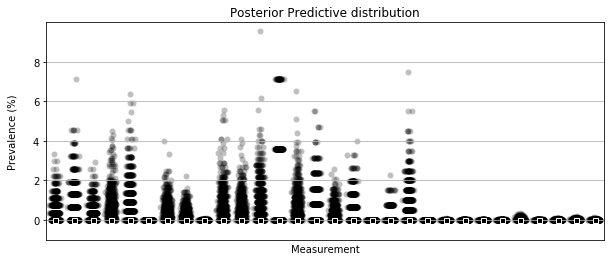Four additional features of DisMod-MR that are important for many settings are:

• informative priors
• fixed effects to cross-walk between different studies
• fixed effects to predict out of sample
• fixed effects to explain the level of variation

Informative priors are useful for modeling disease with less data available than PD, for example to include information that prevalence is zero for youngest ages, or than prevalence must be increasing as a function of age between certain ages.

The informative priors are also key to the "empirical Bayes" approach to modeling age-specific differences between difference GBD regions. In this setting, a model using all the world's data is used to produce estimates for each region, and these estimates are used as priors in region-specific models together with the data relevant to that region only.

"Cross-walk" fixed effects can correct for biases introduced by multiple outcome measures. For example, in the PD dataset,

In :
model = dismod_mr.data.load('pd_sim_data')

In :
crosswalks = list(model.input_data.filter(like='x_cv').columns)
groups = model.get_data('p').groupby(crosswalks)

In :
crosswalks

Out:
['x_cv_ascertainment', 'x_cv_diagnostic_criteria', 'x_cv_representative']
In :
np.round(groups['value'].describe(),3).unstack()['mean'].fillna('-')

Out:
x_cv_representative 0 1
x_cv_ascertainment x_cv_diagnostic_criteria
0 0 0.006 -
1 0.009 -
1 0 0.004 -
1 0.004 0.009

## Incorporating data on parameters other than prevalence¶

So far this example has focused on modeling the prevalence of PD from the prevalence data alone. However, this represents about half of the available data. There is also information on incidence, SMR, and CSMR, which has not yet been incorporated.

DisMod-MR is capable of including all of the available data, using a compartmental model of disease moving through a population. This model formalizes the observation that prevalent cases must once have been incident cases, and continue to be prevalent cases until remission or death.

In this model, incidence, remission, and excess-mortality are age-standardizing negative binomial random effect spline models, while prevalence, SMR, CSMR, and other parameters come from the solution to a system of ordinary differential equations.

The results of this model are smoother prevalence curves that take longer to calculate.

In :
plt.figure(figsize=(10,6))

plt.subplot(2,2,1); dismod_mr.plot.data_bars(model.get_data('p')); plt.xlabel('Age (years)'); plt.ylabel('Prevalence')
plt.subplot(2,2,2); dismod_mr.plot.data_bars(model.get_data('i')); plt.xlabel('Age (years)'); plt.ylabel('Incidence')
plt.subplot(2,2,3); dismod_mr.plot.data_bars(model.get_data('csmr')); plt.xlabel('Age (years)'); plt.ylabel('Cause-specific mortality')
plt.subplot(2,2,4); dismod_mr.plot.data_bars(model.get_data('smr')); plt.xlabel('Age (years)'); plt.ylabel('Standardized \nmortality ratio');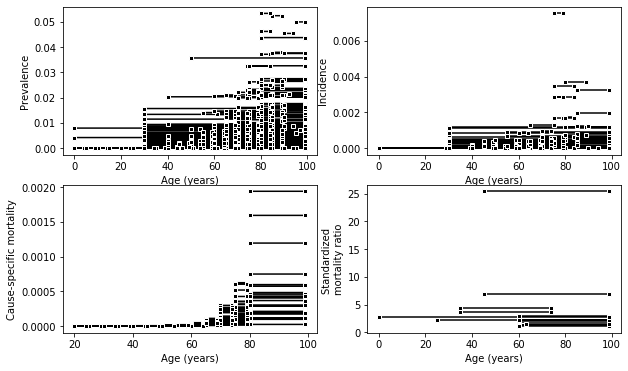In :
model.input_data.columns

Out:
Index(['index', 'age_end', 'age_start', 'age_weights', 'area', 'data_type',
'effective_sample_size', 'lower_ci', 'sex', 'standard_error',
'upper_ci', 'value', 'x_cv_ascertainment', 'x_cv_diagnostic_criteria',
'x_cv_representative', 'year_end', 'year_start'],
dtype='object')
In :
model.vars += dismod_mr.model.consistent(model)
%time dismod_mr.fit.consistent(model)

using stored FE for beta_i_x_cv_ascertainment x_cv_ascertainment {'mu': 0, 'dist': 'Normal', 'sigma': 0.0001}
using stored FE for beta_i_x_cv_diagnostic_criteria x_cv_diagnostic_criteria {'mu': 0, 'dist': 'Normal', 'sigma': 0.0001}
using stored FE for beta_i_x_cv_representative x_cv_representative {'mu': 0, 'dist': 'Normal', 'sigma': 0.0001}
using stored FE for beta_i_x_sex x_sex {'mu': 0, 'dist': 'Normal', 'sigma': 0.0001}
using stored FE for beta_r_x_cv_ascertainment x_cv_ascertainment {'mu': 0, 'dist': 'Normal', 'sigma': 0.0001}
using stored FE for beta_r_x_cv_diagnostic_criteria x_cv_diagnostic_criteria {'mu': 0, 'dist': 'Normal', 'sigma': 0.0001}
using stored FE for beta_r_x_cv_representative x_cv_representative {'mu': 0, 'dist': 'Normal', 'sigma': 0.0001}
using stored FE for beta_r_x_sex x_sex {'mu': 0, 'dist': 'Normal', 'sigma': 0.0001}
using stored FE for beta_f_x_cv_ascertainment x_cv_ascertainment {'mu': 0, 'dist': 'Normal', 'sigma': 0.0001}
using stored FE for beta_f_x_cv_diagnostic_criteria x_cv_diagnostic_criteria {'mu': 0, 'dist': 'Normal', 'sigma': 0.0001}
using stored FE for beta_f_x_cv_representative x_cv_representative {'mu': 0, 'dist': 'Normal', 'sigma': 0.0001}
using stored FE for beta_f_x_sex x_sex {'mu': 0, 'dist': 'Normal', 'sigma': 0.0001}
WARNING: 5 rows of p data has invalid quantification of uncertainty.
using stored FE for beta_X_x_cv_ascertainment x_cv_ascertainment {'mu': 0, 'dist': 'Normal', 'sigma': 0.0001}
using stored FE for beta_X_x_cv_diagnostic_criteria x_cv_diagnostic_criteria {'mu': 0, 'dist': 'Normal', 'sigma': 0.0001}
using stored FE for beta_X_x_cv_representative x_cv_representative {'mu': 0, 'dist': 'Normal', 'sigma': 0.0001}
using stored FE for beta_X_x_sex x_sex {'mu': 0, 'dist': 'Normal', 'sigma': 0.0001}
fitting submodels

/ihme/homes/abie/.local/lib/python3.6/site-packages/pandas/core/indexing.py:480: SettingWithCopyWarning:
A value is trying to be set on a copy of a slice from a DataFrame.
Try using .loc[row_indexer,col_indexer] = value instead

See the caveats in the documentation: http://pandas.pydata.org/pandas-docs/stable/user_guide/indexing.html#returning-a-view-versus-a-copy
self.obj[item] = s

. . . . . . . . . . . . . . . . . . . . . . . . . . . . . . . .
fitting all stochs

finding step covariances
. . . . . . . . . . . . . . . . . . . . . . . . .
sampling from posterior distribution


/homes/abie/.conda/envs/dismod_mr/lib/python3.6/site-packages/pymc/StepMethods.py:1282: UserWarning:
Covariance was not positive definite and proposal_sd cannot be computed by
Cholesky decomposition. The next jumps will be based on the last
valid covariance matrix. This situation may have arisen because no
jumps were accepted during the last interval. One solution is to
increase the interval, or specify an initial covariance matrix with
a smaller variance. For this simulation, each time a similar error
occurs, proposal_sd will be reduced by a factor .9 to reduce the
jumps and increase the likelihood of accepted jumps.

CPU times: user 43min 20s, sys: 53min 23s, total: 1h 36min 44s
Wall time: 30min 50s

Out:
(<pymc.NormalApproximation.MAP at 0x7f7465467198>,
<pymc.MCMC.MCMC at 0x7f746528ebe0>)
In :
plt.figure(figsize=(10,6))

plt.subplot(2,2,1); dismod_mr.plot.data_bars(model.get_data('p')); plt.xlabel('Age (years)'); plt.ylabel('Prevalence')
plt.subplot(2,2,2); dismod_mr.plot.data_bars(model.get_data('i')); plt.xlabel('Age (years)'); plt.ylabel('Incidence')
plt.subplot(2,2,3); dismod_mr.plot.data_bars(model.get_data('csmr')); plt.xlabel('Age (years)'); plt.ylabel('Cause-specific mortality')
plt.subplot(2,2,4); dismod_mr.plot.data_bars(model.get_data('smr')); plt.xlabel('Age (years)'); plt.ylabel('Standardized \nmortality ratio')
param_list = [dict(type='p', title='(a)', ylabel='Prevalence (%)', yticks=([0, .01, .02], [0, 1, 2]), axis=[30,101,-0.001,.025]),
dict(type='i', title='(b)', ylabel='Incidence \n(per 1000 PY)', yticks=([0, .001,.002, .003, .004], [0, 1, 2, 3, 4]), axis=[30,104,-.0003,.0055]),
dict(type='pf', title='(c)', ylabel='Cause-specific mortality \n(per 1000 PY)', yticks=([0, .001,.002], [0, 1, 2]), axis=[30,104,-.0002,.003]),
dict(type='smr', title='(d)', ylabel='Standardized \nmortality ratio', yticks=([1, 2, 3,4, ], [1, 2,3, 4]), axis=[35,104,.3,4.5]),
]

for i, params in enumerate(param_list):
ax = plt.subplot(2,2,i+1)
if params['type'] == 'pf': dismod_mr.plot.data_bars(model.get_data('csmr'), color='grey')
else: dismod_mr.plot.data_bars(model.get_data(params['type']), color='grey')

if params['type'] == 'smr': model.pred = dismod_mr.model.predict_for(model, model.parameters.get('smr', {}), 'all', 'female', 2005,
'GBR', 'female', 2005, 1., model.vars['smr'], 0., 100.).T
else : model.pred = dismod_mr.model.predict_for(model, model.parameters[params['type']],
'all', 'female', 2005,
'GBR', 'female', 2005, 1., model.vars[params['type']], 0., 1.).T

plt.plot(np.arange(101), model.pred.mean(axis=1), 'k-', linewidth=2, label='Posterior Mean')
hpd = mc.utils.hpd(model.pred.T, .05)
plt.plot(np.arange(101), hpd, 'k-', linewidth=1, label='95% HPD interval')
plt.plot(np.arange(101), hpd, 'k-', linewidth=1)

plt.xlabel('Age (years)')
plt.ylabel(params['ylabel']+'\n\n', ha='center')
plt.axis(params.get('axis', [-5,105,-.005,.06]))
plt.yticks(*params.get('yticks', ([0, .025, .05], [0, 2.5, 5])))
plt.title(params['title'])
plt.grid()


/homes/abie/.conda/envs/dismod_mr/lib/python3.6/site-packages/ipykernel_launcher.py:14: MatplotlibDeprecationWarning: Adding an axes using the same arguments as a previous axes currently reuses the earlier instance.  In a future version, a new instance will always be created and returned.  Meanwhile, this warning can be suppressed, and the future behavior ensured, by passing a unique label to each axes instance.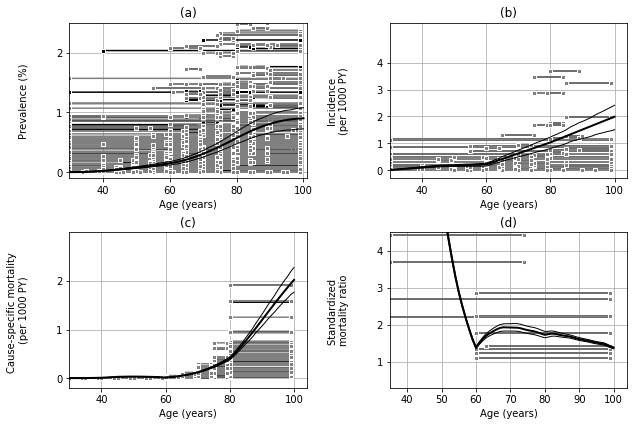In :
p_with = model


The most notable difference between the estimates from this model and from the model that used prevalence data only is that this model produces estimates of incidence and mortality in addition to prevalence. In many cases, the model also produces estimates of the remission rate as well, but there is no remission of PD, so the estimates of zero are not very interesting in this example. It is another place that informative priors are useful, however.

There are also differences between the means and uncertainty intervals estimated by these methods, which show that the additional data is important. Although the prevalence data alone predicts age-specific prevalence that peaks at 2%, when the incidence and mortality data is also included, the maximum prevalence is a bit lower, closer to 1.5%.

In :
p1 = dismod_mr.model.predict_for(p_only, model.parameters['p'],
'all', 'total', 'all',
'GBR', 'female', 2005, 1.,
p_only.vars['p'], 0., 1.)

p2 = dismod_mr.model.predict_for(p_with, model.parameters['p'],
'all', 'total', 'all',
'GBR', 'female', 2005, 1.,
p_with.vars['p'], 0., 1.)

In :
plt.plot(p1.mean(axis=0), 'k--', linewidth=2, label='Only prevalence')
plt.plot(p2.mean(axis=0), 'k-', linewidth=2, label='All available')

plt.xlabel('Age (years)')
plt.ylabel('Prevalence (%)\n\n', ha='center')
plt.yticks([0, .01, .02], [0, 1, 2])
plt.axis([30,101,-0.001,.025])
plt.legend(loc='upper left')
plt.grid()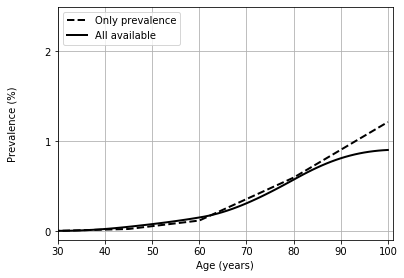Because the data is so noisy, the differences between the mean estimates of these different models are not significant; the posterior distributions have considerable overlap. At age 80, for example, the posterior distributions for age-80 prevalence are estimated as the following:

In :
plt.hist(100*p1[:,80], density=True, histtype='step', label='Only prevalence', linewidth=3, color=np.array([239., 138., 98., 256.])/256)
plt.hist(100*p2[:,80], density=True, histtype='step', label='All available', linewidth=3, color=np.array([103, 169, 207, 256.])/256)
plt.title('PD prevalence at age 80')
plt.xlabel('Prevalence (%)\n\n', ha='center')
plt.ylabel('Probability Density')
plt.legend(loc='upper right')
plt.grid()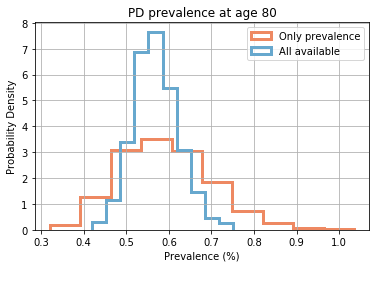# Conclusion¶

I hope that this example is a quick way to see the strengths and weaknesses of DisMod-MR. This model is particularly suited for estimating descriptive epidemiology of diseases with sparse, noisy data from multiple, incompatible sources.

I am currently working to make it faster, as well as to improve the capabilities for modeling changes between regions over time.

In :
!date

Tue Jul 23 14:29:20 PDT 2019